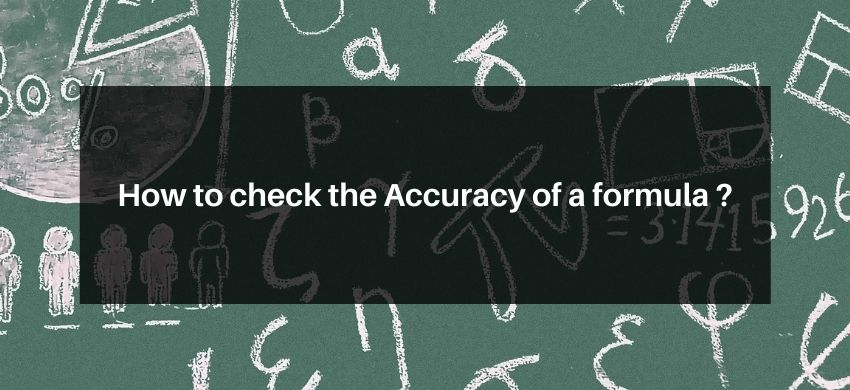# How to check the Accuracy of a formula ?## To check the accuracy of a formula

It is based on the homogeneity principle of dimension according to it formula is correct when L.H.S. $=$ R.H.S. Dimensionally.

Ex. Test the correctness of the formula $\mathrm{T}=2 \pi \sqrt{\frac{\ell}{\mathrm{g}}}$, where, $\mathrm{T}=$ time period $\ell=$ length of pendulum and $\mathrm{g}=$ Acc. due to gravity.

Sol. L.H.S. Dimension Equation of $\mathrm{T} \Rightarrow \mathrm{M}^{0} \mathrm{~L}^{0} \mathrm{~T}^{1}$

R.H.S. Dimension equation of; $2 \pi \sqrt{\frac{\ell}{g}} \Rightarrow\left[\frac{M^{0} L^{1} T^{0}}{L^{1} T^{-2}}\right]^{1 / 2}=\left[M^{0} L^{0} T^{2}\right]^{1 / 2}=M^{0} L^{0} T^{1}$

$\because$ L.H.S. = R.H.S.; Dimensionally. Therefore, the given formula is correct.

Some Important problems based on homogeneity Theory of Dimension

1. Experiment suggests the velocity of a sphere allowed to fall from rest in the viscous medium increased exponentially as $v=v_{0}\left(1-e^{-k t}\right)$. Evaluate the dimension of 'K'

2. Find the dimension $R, a$ and $b$ in the vander wals formula $\left(p+\frac{a}{V^{2}}\right)(V-b)=R T$, Where, $p=$ pressure, $\mathrm{T}=$ Temp. and $\mathrm{V}$ is volume.

3. In the formula $\mathrm{p}=\frac{\mathrm{RT}}{\mathrm{V}-\mathrm{b}} \mathrm{e}^{-\frac{\mathrm{a}}{\mathrm{RTV}}}$, find the dimension of a and $\mathrm{b}$.

4. The charge on a capacitor is found to increase expotentially as $q=q_{0}\left(1-e^{-k t}\right) .$ Find the value of $k$ in terms of resistance $(R)$ and capacitance( $c$ ).